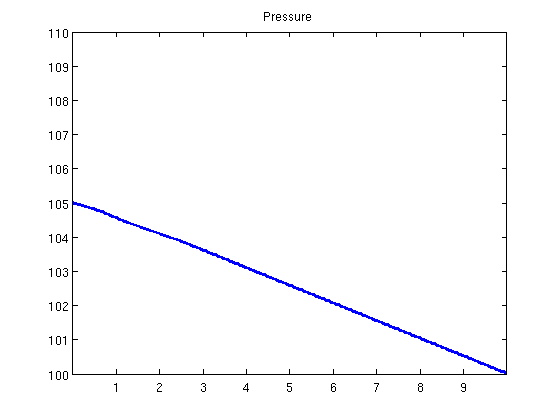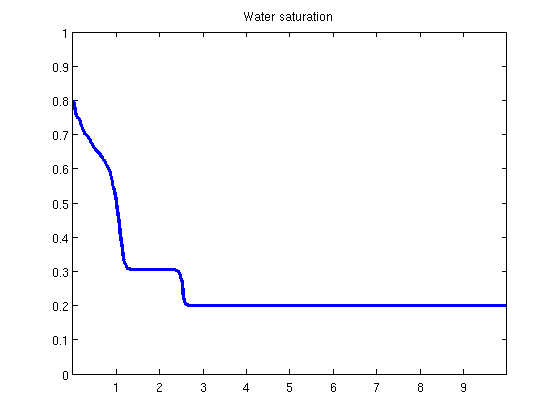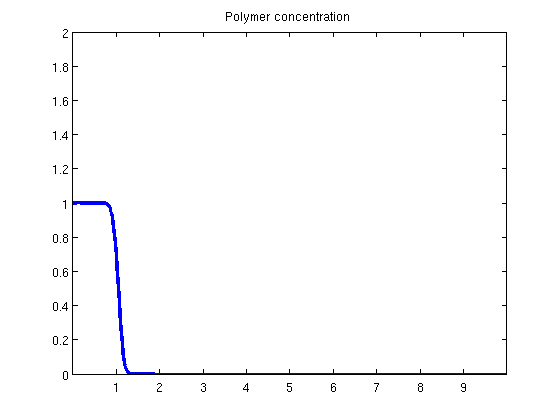#Function runLabPolymer.m

## Simple Polymer simulator

We set up a simple polymer simulator with a cartesian grid and physical boundary conditons such as given influx in input cells and given pressure, saturation and concentration on output faces.

```function [G, states] = runLabPolymer()
```

Add mrst modules which are used

```   mrstModule add ad-fi deckformat mrst-gui ad-props
```

Input data are read from a file. The format is the same as Eclipse. Values are store in the deck structure. The input file contains the fluid and polymer properties.

```   current_dir = fileparts(mfilename('fullpath'));
fn = fullfile(current_dir, 'LABPOLYMER.DATA');
```

The deck variables are converted to SI units. MRST uses exclusively SI units in the computation. Conversion tools are available.

```   deck = convertDeckUnits(deck);
```

Generate grid. We set up two cases: 1D or 2D. The changes in the remaining of the code are minimal. They appear only in the file setupControls.m where the boundary conditions are set.

```   sim_case = '1D'; % '1D' or '2D'

switch sim_case

case '1D'

nx = 1000;
ny = 1;
nz = 1;

xlength = 10;
ylength = 1;
zlength = 1;

case '2D'

nx = 100;
ny = 100;
nz = 1;

xlength = 10;
ylength = 10;
zlength = 1;

end
```

The function ¦cartGrid¦ generates a MRST unstructured grid. The function computeGeometry computes geometrical properties such as cell volumes, faces areas...

```   G = cartGrid([nx ny nz], [xlength, ylength, zlength]);
G = computeGeometry(G);
```

Setup rock structure containing the rock properties.

```   rock.perm = repmat(100*milli*darcy, [G.cells.num, 1]);
rock.poro = repmat(0.3, [G.cells.num, 1]);
```

Setup fluid structure containing the fluid and polymer properties form the deck structure.

```   fluid = initDeckADIFluid(deck);
```

We set off gravity.

```   gravity off
```

Setup adsorption function. There are two options: with or without desorption.

```   fluid.effads = @(c, cmax) effads(c, cmax, fluid);
```

Setup the inputs. They are given as boundary conditions and sources

Injection will done in given cells.

```   bc.injection.rate = 0.1/day;
bc.injection.s = 1;
bc.injection.c = 1;
```

pressure, saturation and polymer concentration are imposed on given faces

```   bc.dirichlet.pressure = 100*barsa;
bc.dirichlet.s = 0;
bc.dirichlet.c = 0;
```

The function setupControls.m set up the bc structure.

```   bc = setupControls(G, bc, sim_case);
```

The function setupSystem.m sets up the system. In particular, it defines the discrete differential operators.

```   system = setupSystem(G, rock, bc);
system.fluid = fluid;
system.rock = rock;
system.nonlinear.tol           = 1e-4;
system.nonlinear.maxIterations = 30;
system.nonlinear.relaxRelTol   = 0.2;
system.cellwise                = 1:3;
```

The transport part can be either solved explicitly or implicitly. From the implementation point of view, it corresponds to one line of change in the code, see equationOWPolymer.m

```   system.implicit_transport = false;
```

Set up the initial state with constant pressure, saturation and polymer concentration.

```   nc = G.cells.num;
init_state.pressure = 1*atm*ones(nc,1);
init_state.s        = ones(nc, 1)*[0.2, 0.8];
init_state.c        = zeros(G.cells.num, 1);
init_state.cmax     = zeros(G.cells.num, 1);
```

Compute and store the mobilities for the Dirichlet values

```   [bc.mobW, bc.mobO, bc.mobP] = computeMobilities(bc.dirichlet.pressure, ...
bc.dirichlet.s       , ...
bc.dirichlet.c       , ...
bc.dirichlet.c       , ...
fluid);
```

Set up time stepping parameters. We use a fixed time step.

```   total_time = 2*day;
dt         = 0.001*day;
steps      = dt*ones(floor(total_time/dt), 1);
t          = cumsum(steps);
```

We start the time step iterations. Each iteration is solved fully implicitly.

Time step iterations.

```   state0 = init_state;

nsteps = numel(steps);
states = cell(nsteps, 1);

for tstep = 1 : nsteps
```
```      dt = steps(tstep);
```

Call non-linear solver solvefi.m to compute next state

```      [state, conv, its] = solvefi(state0, dt, bc, system);

if ~(conv)
error('Convergence failed. Try smaller time steps.')
return
else
fprintf('Step %d completed in %d Newton iterations\n', tstep, its);
end

states{tstep} = state;
state0 = state;
```
```   end

plotState(state, G.cells.centroids(:, 1));
``````end
```

## Helper function

```function y = effads(c, cmax, f)
else
end
end

function plotState(state, xval)
figure(1)
plot(xval, state.pressure/barsa);
axis([xval(1), xval(end), 100, 110]);
title('Pressure')
figure(2)
plot(xval, state.s(:, 1));
axis([xval(1), xval(end), 0, 1]);
title('Water saturation')
figure(3)
plot(xval, state.c);
axis([xval(1), xval(end), 0, 2]);
title('Polymer concentration')
end
```

Published November 6, 2014